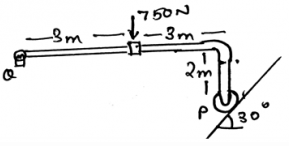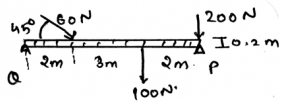Courses

# Test: Stability Of Equilibrium Configurations

## 15 Questions MCQ Test Engineering Mechanics | Test: Stability Of Equilibrium Configurations

Description
This mock test of Test: Stability Of Equilibrium Configurations for Mechanical Engineering helps you for every Mechanical Engineering entrance exam. This contains 15 Multiple Choice Questions for Mechanical Engineering Test: Stability Of Equilibrium Configurations (mcq) to study with solutions a complete question bank. The solved questions answers in this Test: Stability Of Equilibrium Configurations quiz give you a good mix of easy questions and tough questions. Mechanical Engineering students definitely take this Test: Stability Of Equilibrium Configurations exercise for a better result in the exam. You can find other Test: Stability Of Equilibrium Configurations extra questions, long questions & short questions for Mechanical Engineering on EduRev as well by searching above.
QUESTION: 1

### The main condition for the stability of equilibrium configuration is that the distance between various particles of the body does change.

Solution:

The main condition for the rigid body is that the distance between various particles of the body does not change. If the distance does change the body is not to be called as rigid. Thus the fix distance of the particles is very much required for the equilibrium of the rigid body.

QUESTION: 2

### Determine the vertical components of the reaction on the beam caused by the pin at Q.Solution:

The summation of the forces needs to be zero. So does the summation of the moments need to zero. But talking about the angles, they not needed to zero. But the forces which are acting at particular angles, must needed to be equal to zero. The basic need of the forces to make the body in equilibrium.

QUESTION: 3

### Which of the following is correct for stability of equilibrium configuration?

Solution:

The application of the conditions of the equilibrium of the body is valid throughout. This means that the conditions are irrespective of the dimensions. The conditions are the basic rules that defines the equilibrium of the body and thus are applicable in any dimension of the real axis.

QUESTION: 4

For stability of equilibrium configuration the net force acting on the body is zero.

Solution:

The equilibrium is only attained if the net force on the body tends to be equal to zero. Thus the forces cancels out. If this happens there is no motion of the body along any direction and hence the body is said to be in equilibrium. The body here is a rigid body.

QUESTION: 5

For stability of equilibrium configuration the net moment acting on the body by various forces is zero.

Solution:

The equilibrium is only attained if the net moment on the body tends to be equal to zero. Thus the moments caused by different forces cancels out. If this happens there is no motion of the body along any direction and hence the body is said to be in equilibrium. The body here is a rigid body.

QUESTION: 6

The net force of the body is zero that means the force are not being applied to the body at all and hence the body has achieved stability.

Solution:

The net force of the body is zero that doesn’t means that the force are not being applied to the body at all and hence the body is in equilibrium. The equilibrium is only attained if the net force on the body tends to be equal to zero. Thus the forces cancels out. If this happens there is no motion of the body along any direction and hence the body is said to be in equilibrium.

QUESTION: 7

Which of the following needs to zero for the stability of equilibrium configuration?

Solution:

The summation of the forces needs to be zero. So does the summation of the moments need to zero. But talking about the angles, they not needed to zero. But the forces which are acting at particular angles, must needed to be equal to zero. The basic need of the forces to make the body in equilibrium.

QUESTION: 8

Determine the horizontal components of the reaction on the beam caused by the pin at Q. The force 60N is multiplied by 10 and then is applied.Solution:

The summation of the forces needs to be zero. So does the summation of the moments need to zero. But talking about the angles, they not needed to zero. But the forces which are acting at particular angles, must needed to.

QUESTION: 9

Free body diagrams doesn’t play any role in making the calculations on the conditions of stability of equilibrium configuration.

Solution:

The free body diagrams does play an important role in the formation of the conditions of the equilibrium of the rigid body. As the net forces are zero, the fbd helps us to take the measure of the same. That is to see whether the summation is really zero or not.

QUESTION: 10

The net moment of the body is zero that means the distance between the force and the rotational axis is zero thus the stability of equilibrium configuration.

Solution:

The net moment of the body is zero that doesn’t means that the distance between the force and the rotational axis is zero. This means moments caused by different forces cancels out. If this happens there is no motion of the body along any direction and hence the body is said to be in equilibrium.

QUESTION: 11

If the resolved force for the stability of equilibrium configuration or the force which you get as the answer after solving the question is negative, then what does this implies?

Solution:

The negative sign implies things in the opposite manner. If the force is coming negative this doesn’t means that it is impossible. But it means that the force is in the opposite direction w.r.t the direction set by you in the free body diagram.

QUESTION: 12

If the body is in stability of equilibrium configuration, but it having a rotational curled ray shown in the free body diagram then:

Solution:

The body having equilibrium will not rotate at any cost. Yes, the diagram may contain the rotational array showing the couple being acted over the structure. But the thing is that the forces, i.e. the other forces which are outside the dependency of this rotation will cancel out this rotation and thus the body is in equilibrium.

QUESTION: 13

If five forces are acting on the single particle and having an angle of 72˚ between each and are collinear, then:

Solution:

The net force acting on the body is zero. This means that the forces cancel out. This means that the body is in equilibrium and doesn’t need any of the external force to make itself in the equilibrium.

QUESTION: 14

For the conditions of the stability of equilibrium configuration of the body, i.e. the body only the external forces defines the equilibrium. Because the internal forces cancels out so not to be considered.

Solution:

The application of the internal forces does affect the conditions of the equilibrium of the body. Not only the external but the internal forces that are developed by the sake of external forces does develop a tending effect on the equilibrium of the body. Thus the internal forces doesn’t cancels out.

QUESTION: 15

We need to apply vector math sometimes in the calculation of stability of equilibrium configurations. So the coordinate of the Force vector AB is A (2, 0, 2) and B (-2, 3.46, 3). It has a magnitude of 750N. Which is the best Cartesian representation of the vector AB?

Solution:

First try to make the unit vector of AB. Then you will get the Cartesian form of unit vector, after that just multiply 750 scalar quantity with it. Which means you are first taking out the desired direction and then multiplying the scalar to get the desired vector in desired direction.